Latest Banking jobs   »   IBPS quant

# Quantitative Aptitude Quiz For IBPS RRB PO/Clerk Mains 2023-02nd-September

Direction (1-5): Read the given information carefully and answer the following questions. The given graph shows the profit percentage of three companies in different years.
Profit= Income- Expenditure and profit percentage is calculated using income as a reference.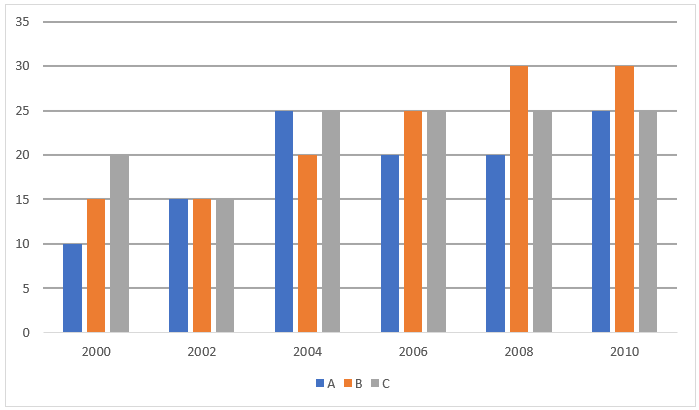Q1. What is the difference (in Rs. Lakhs) between the profits of A in 2000 and 2002? Assume that the expenditures of A in 2000 and 2002 were Rs. 9 lakhs and Rs. 10.2 lakhs respectively.
(a) Rs 72,000
(b) Rs 60,000
(c) Rs 75,000
(d) Rs 80,000
(e) Rs 90,000

Q2. The expenditure of B in 2004 was same as the expenditure of C in 2000, what was the ratio of the income of B in 2004 to that of C in 2000?
(a) 3: 2
(b) 1: 1
(c) 5: 4
(d) 2: 3
(e) None of these

Q3. The ratio of the incomes of A and B in 2008 was 5: 4. What was the ratio of the expenditure of A to that of B in that year?
(a) 10: 7
(b) 10: 9
(c) 5: 4
(d) 3: 2
(e) 6: 5

Q4. If the expenditure of A in 2002 was Rs 50 lakh and that of C and B together in that year is Rs 20 lakhs more than that of A, then what was the ratio of the income of A to that of B and C together?
(a) 4: 7
(b) 5: 8
(c) 5: 7
(d) 2: 3
(e) 5: 6

Q5. The sum of income of C in 2006 and that of B in 2010 is Rs 15 lakh and expenditure of B in 2010 is Rs 1.8 lakhs more than the expenditure of C in 2006 then find the difference of their income in the given year?
(a) 4.2 lakhs
(b) 4 lakhs
(c) 2.5 lakhs
(d) 3 lakhs
(e) 3.4 lakhs
Directions (6-10): Bar Graph gives information about no. of books of Maths, English, and G.S in five different years. Use the information to give answer of following questions.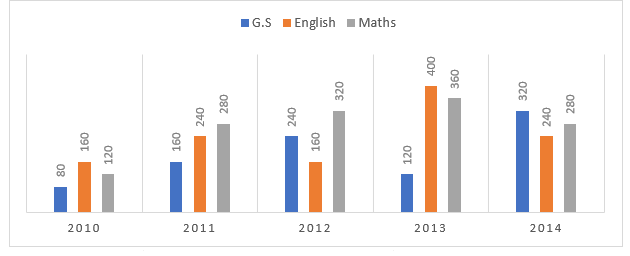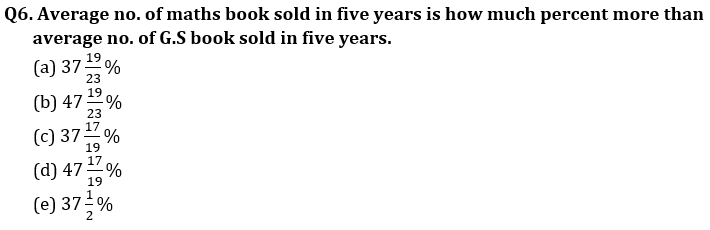Q7. In which year average no. of book sold is 100% more than average no. of book sold in 2010.
(a) 2011
(b) 2013
(c) 2012
(d) 2014
(e) None of these

Q8. Total no. of book sold in 2011 is how much percent more than average no. of maths book sold in five years.
(a) 150 %
(b) 100 %
(c) 120 %
(d) 105 %
(e) 110 %

Q9. What is the ratio of total no. of book sold of G.S in 2011, English in 2012, Maths in 2013 to total no. of books sold of G.S in 2014, English in 2010, and Maths in 2010.
(a) 17 : 18
(b) 15 : 16
(c) 15 : 17
(d) 17 : 15
(e) 16 : 17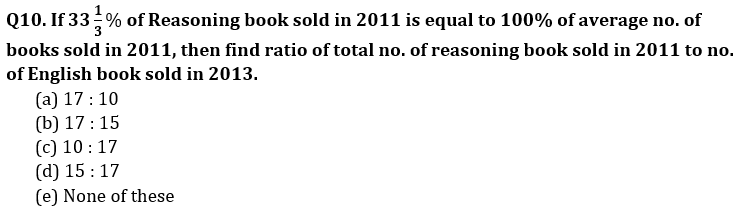Solutions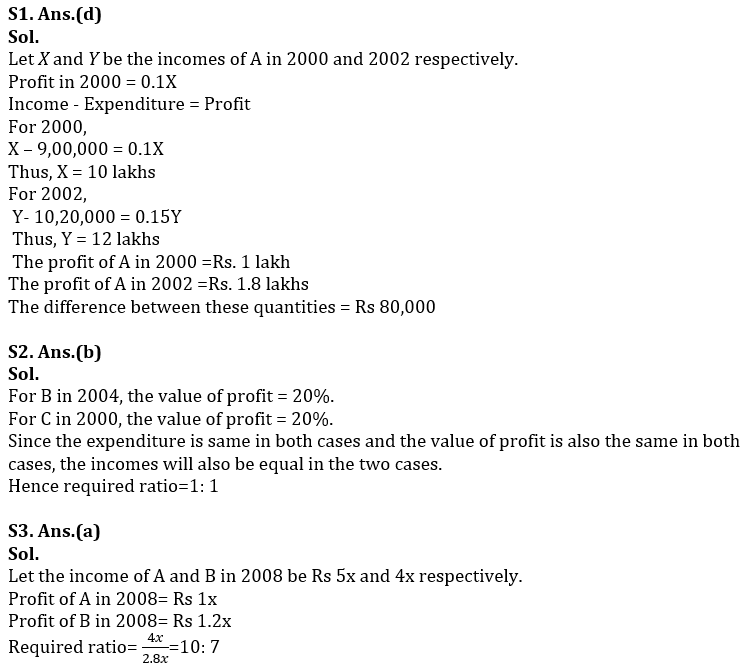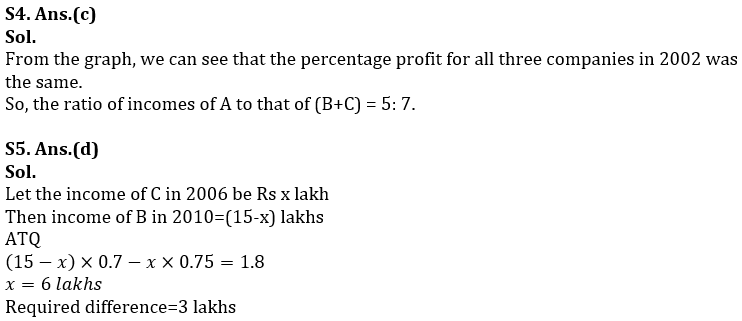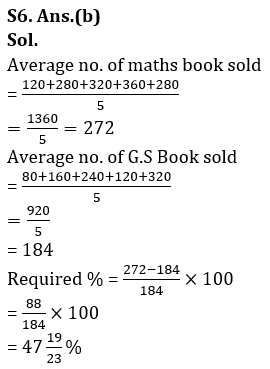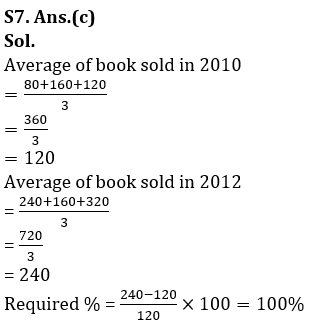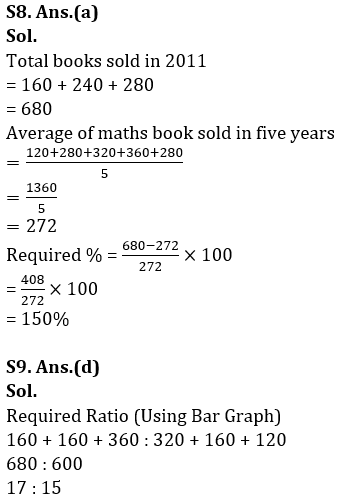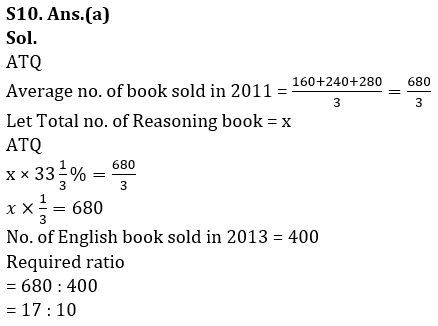## FAQs

### When will the IBPS RRB PO mains exam be conducted?

IBPS RRB PO mains exam will be conducted on 10 September 2023

#### Congratulations!Union Budget 2023-24: Free PDF